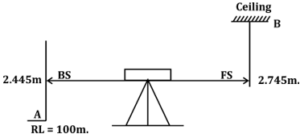Engineering Jobs   »   Civil Engineering Quiz

# GATE’22 CE:- Daily Practice Quiz 30-Aug-2021

IIT Kharagpur has released the GATE 2022 exam dates. As per the GATE 2022 exam date, the exam will be conducted on February 5, 6, 12 & 13. Candidates preparing for GATE 2022 must attempt this Civil Engineering Quiz to boost your GATE exam preparation.

Quiz: Civil Engineering
Exam: GATE
Topic: Miscellaneous

Each question carries 2 marks
Negative marking: 1/3 mark
Time: 12 Minutes

Q1. If density of water is 1000 kg/m³ gravitational acceleration is 9.81 m/sec² and the specific gravity of liquid is 0.8, the pressure head in terms of height of liquid column for pressure intensity of 1 kg/cm² is equal to
(a) 21.5m
(b) 7.5m
(c) 10m
(d) 12.5m

Q2. The R.L. of the point ‘A’ which is one floor is 100 m and back sight reading on ‘A’ is 2.445m. if the foresight reding on the point ‘B’ which is on ceiling is 2.745m the R.L. of point ‘B’ will be –
(a) 94.80 m
(b) 99.71 m
(c) 100.29 m
(d) 105.20 m

Q3. The dimensions for a rectangular primary sedimentation tank are, length = 30m; breadth = 10m and depth = 3m. if the flow rate through the tank is 0.1 m³/s, the surface overflow rate (SOR) of tank is ______________.
(a) 14.4 m³/m²/day
(b) 28.8 m³/m²/day
(c) 9.6 m³/m²/day
(d) 21.6 m³/m²/day

Q4. The maximum deflection in timber beams or joints should not be greater than
(a) Span/300
(b) Span/325
(c) Span/360
(d) Span/380

Q5. The following data were obtained when a sample of medium sand was tested in a constant head permeameter:
Cross-section area of sample : 100 cm²
Discharge collected : 10 cc/s
The coefficient of permeability of the sand is
(a) 0.1 m/s
(b) 0.01 m/s
(c) 1 × 10^(-4) m/s
(d) 1 × 10^(-8) m/s

Solutions

S1. Ans.(d)
Sol. Given,
ρ_w=10^3 kg\/m^3
g = 9.81 m/sec²
S_l=0.8
ρ_l=0.8 × 10³ kg/m³
P = 1 kg/cm²= 10^4 kg\/m^2
h= ?
We know,
P = ρ_l gh
10^4= 0.8 × 10³ × 9.81 × h
▭(h=12.5m)

S2. Ans.(d)
Sol.H.I = RL + BS
= 100 + 2.445
= 102.445m.
RL at point B = HI – (FS)
= 102.445 – (–2.745)
= 105.19 ≈ 105.20m.

S3. Ans.(b)
Sol. Given,
Flow rate (Q) = 0.1 m³/s.
Length (L) = 30m.
Depth (D) = 3m.
Surface overflow rate (SOR) = ?
SOR = ▭((Flow rate (Q))/(Surface Area (B.L) ))
= (0.1×24×3600)/(10×30)
▭(SOR=28.8 m^3 \/m^2 \/day)

S4. Ans.(c)
Sol. As per IS 883:1994, The deflection in case of battens, purlins, beam joist and other flexural members supporting brittle material shall not be greater than span/360.

S5. Ans.(c)
Sol. Given,
Q = 10 cc/sec.
i = 10
A = 100 cm²
K = ?
We know,
Q = KiA
10 = K × 10 × 100
K=1/100 cm\/sec⁡
▭(K=1×10^(-4) m\/sec.)

Sharing is caring!

Thank You, Your details have been submitted we will get back to you.